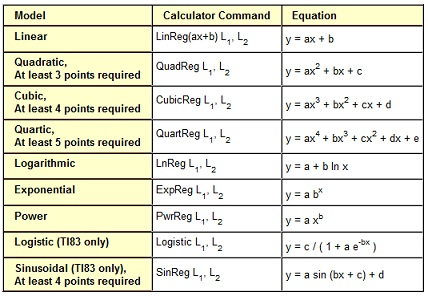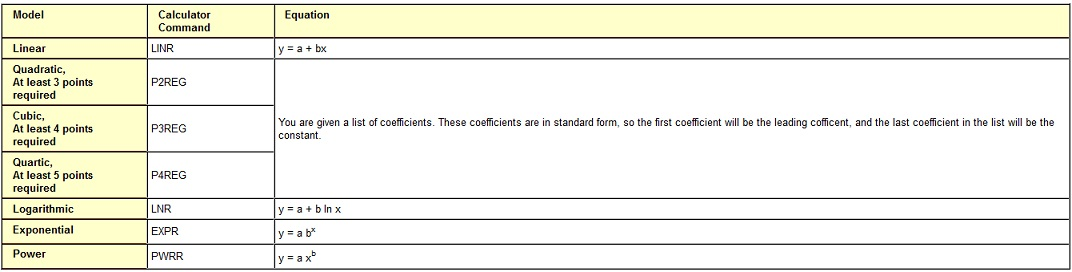#### Exploring Data: Nonlinear Models

Exploring Data: Nonlinear Models:

TI-82/TI-83 Models:With TI-83, you might store the regression equation directly to the y-variable by specifying the y-variable after the lists where your data is stored. Illustration: ‘LnReg L1, L2, y1’ would calculate the logarithmic model and save the outcome into y1.

TI-85 Models:Note that things are a quite different for TI-85 when it comes to the polynomial regression. The outcomes are not given in the equation form with individual coefficients, however are given as a list of coefficients and stored in variable PRegC. When PRegC = {3, 2, -1, 5}, then the regression equation would be y = 3x3 + 2x2 - x + 5. DRREG will properly draw the regression equation even when polynomial regression is utilized.

Least Squares Regression Model:

The concept behind fitting a model to the data is to determine the model which consists of the smallest deviation from real data. This deviation is predicted (predicted by the model) y-value minus the real y-value. Such deviations are all squared to make sure that they do not cancel one other out, and then added. We are then looking for minimum sum of the squared deviations that is the ‘Least Squares’ model.

TI-82/TI-83:

Suppose that you have entered the x-values to the list 1 and the y-values to the list 2. You have calculated whichever model you are working with and have saved the resultant equation to y1.

The expected y-values can be found by computing y1 at each x-value (list 1): y1(L1)
The sum of squared deviations can be found by using the given command:

sum ((L2 - y1(L1))2)

a) The ‘sum’ command can be found beneath the LIST key. After pressing 2nd Stat to obtain the List menu, arrow right to Math, and select sum.

b) The L1 and L2 commands can be obtained by pressing 2nd 1 and 2nd 2.

c) The y-variables similar to y1 can be found on TI-82 beneath the Y-VARS key (2nd VARS), and on TI-83 beneath the VARS key, and then arrow right to the Y-Vars option.

The model with the minimum sum of the squared deviations is the most excellent model.

TI-85:

With TI-85, this is a much tougher task to accomplish. Compute the regression equation as normal, and then enter the given expression to determine the sum of squares (additional spaces inserted for the readability).

sum seq((yStat(x) - fcsty xStat(x))2, x, 1, dimL xStat, 1)

a) Sum, seq, and dimL can be found beneath the LIST key, and OPS sub menu.

b) The xStat and yStat can be found beneath the LIST key, and NAMES sub menu. They can as well be found beneath the STAT key and VARS sub menu, however it is probably simpler from list as that is where the rest of commands are.

c) The fcsty can be found beneath the CATALOG by hitting the Alpha and then F keys.

Correlation Coefficient:

The closer the value of r was to 1 or -1, the better the model fit data. For non-linear models, we frequently avoid the value of r and look at the value of r2. The value of r2 can be found by simply squaring the r.

There is for all time variation in data. That is, never (almost) is all the data precisely the same. The interpretation of r2, from a statistical view point is the percent of variation which can be described by the model. The higher the value of r-squared, better the model describes the data.

The TI-85 doesn’t compute the value of r2 for polynomial (quadratic, cubic or quartic models). For other models, you might have to square the correlation (that is, corr variable). The variables essential to simply calculate the value of correlation coefficient are not calculated whenever doing polynomial regression, therefore finding r or r2 for polynomial regression are going to be very difficult for TI-85 users. Ii is recommended to find a TI-82 or TI-83 and borrow it long adequate to do that specific regression model.
The model with maximum value for r2 is the best model.

Latest technology based Algebra Online Tutoring Assistance

Tutors, at the www.tutorsglobe.com, take pledge to provide full satisfaction and assurance in Algebra help via online tutoring. Students are getting 100% satisfaction by online tutors across the globe. Here you can get homework help for Algebra, project ideas and tutorials. We provide email based Algebra help. You can join us to ask queries 24x7 with live, experienced and qualified online tutors specialized in Algebra. Through Online Tutoring, you would be able to complete your homework or assignments at your home. Tutors at the TutorsGlobe are committed to provide the best quality online tutoring assistance for Algebra Homework help and assignment help services. They use their experience, as they have solved thousands of the Algebra assignments, which may help you to solve your complex issues of Algebra. TutorsGlobe assure for the best quality compliance to your homework. Compromise with quality is not in our dictionary. If we feel that we are not able to provide the homework help as per the deadline or given instruction by the student, we refund the money of the student without any delay.